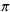Create a new printableGeometry
Math Worksheets

Sample - Click above to make a new math worksheet (PDF).
 Name _____________________________Date ___________________
Perimeter and Area
Find the radius of the circle. (use= 3.14)

 1 * This is a pre-made sheet.Use the link at the top of the page for a printable page.
2.
 A  = 324cm2

3.
 C  = 46cm

4.
 A  = 1929.67 m2

5.
 C  = 15.86mm

6.
 A  = 124.5456cm2

7.
 C  = 3cm

8.
 A  = 81mm2

9.
 C  = 36cm

10.
 C  = 1.71mm

11.
 A  = 1256 mm2

12.
 C  = 9.9mm

13.
 A  = 335.9889m2

14.
 C  = 43.96 cm

15.
 A  = 1256 cm2

16.
 C  = 159.07 m

17.
 A  = 0.03m2

18.
 C  = 40.86m

 19 * This is a pre-made sheet.Use the link at the top of the page for a printable page.
20.
 A  = 1661.06 cm2

21.
 C  = 34.9m

22.
 A  = 289cm2

23.
 C  = 21.1mm

24.
 A  = 907.46 mm2

25.
 C  = 1.14cm

26.
 C  = 81.39 cm

27.
 A  = 2014.65 cm2